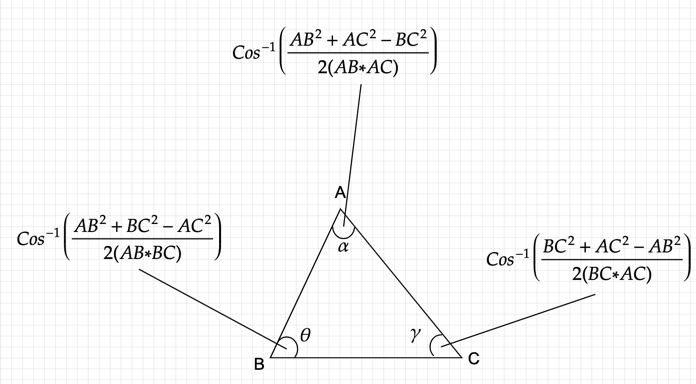# Using the law of cosines and vector dot product formula to find the angle between three points

For any 3 points A, B and C on a cartesian plane. If we have to find the angle between these points, there are many ways we can do that. In this article I will talk about the two frequently used methods:
1. The Law of Cosines formula
2. Vector Dot product formula

## Law of Cosines

For any given triangle ABC with sides AB, BC and AC, the angle formed by the lines AB and BC is given by the formula: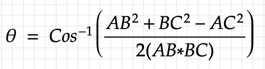Here is how we can derive that formula: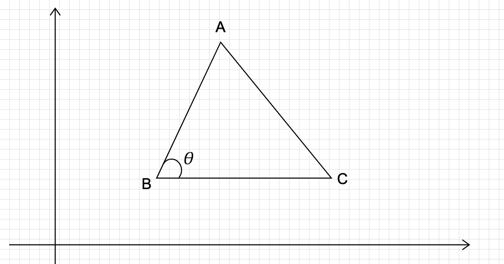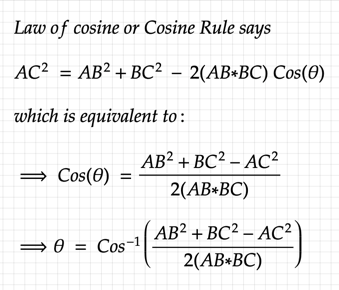The same can be extended for other angles as well.Now, if we were given 3 points on a cartesian plane, we can find the distance between any two points using the Euclidean distance formula: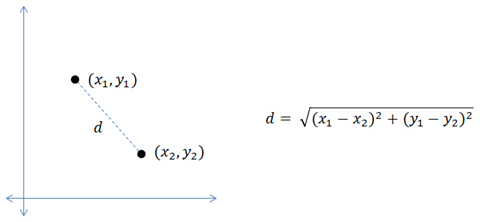So, to find the angle between three points A(x1,y1), B(x2,y2) and C(x3,y3), our formula becomes: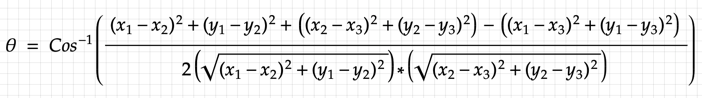In Python we can represent the above formula using the code:

```import numpy as np
import math

def angle_between_three_points(pointA, pointB, pointC):

x1x2s = math.pow((pointA - pointB),2)
x1x3s = math.pow((pointA - pointC),2)
x2x3s = math.pow((pointB - pointC),2)

y1y2s = math.pow((pointA - pointB),2)
y1y3s = math.pow((pointA - pointC),2)
y2y3s = math.pow((pointB - pointC),2)

cosine_angle = np.arccos((x1x2s + y1y2s + x2x3s + y2y3s - x1x3s - y1y3s)/(2*math.sqrt(x1x2s + y1y2s)*math.sqrt(x2x3s + y2y3s)))

return np.degrees(cosine_angle)

A = np.array([2,4])
B = np.array([8,7])
C = np.array([9,1])

print("Angle between points:", angle_between_three_points(A,B,C))
# gives 72.89

A = np.array([0,0])
B = np.array([0,4])
C = np.array([4,0])

print("Angle between points:", angle_between_three_points(A,B,C))
# gives 45.0```

## Vector Dot Product

The three points can also be represented as a vector as shown below.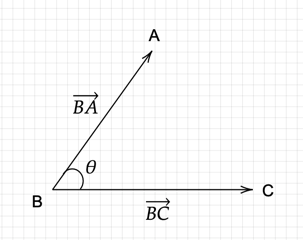where,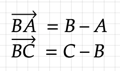The scalar product or the dot product of two vectors is represented by the formula: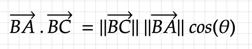where || * || is the magnitude of the vector and θ is the angle made by the two vectors.

From the above formula we can represent the angle using the formula: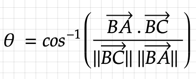In Python we can represent the above formula using the code:

```def angle_between_three_points(pointA, pointB, pointC):
BA = pointA - pointB
BC = pointC - pointB

try:
cosine_angle = np.dot(BA, BC) / (np.linalg.norm(BA) * np.linalg.norm(BC))
angle = np.arccos(cosine_angle)
except:
print("exc")
raise Exception('invalid cosine')

return np.degrees(angle)```

The above is code from my post where I talked about finding the collinearity of three points on a plane using the angle formed by the points.

References: